# What do the AR dashboard metrics mean?

If you want to quickly understand what's happening with your customer accounts, the dashboard is where all of this information is aggregated and displayed. Here we will explain the terms to you so that you can get the most out of the metrics. Also, in case you have invoices in multiple currencies, Peakflo will show the data of all the invoices in the base currency on the accounts receivable dashboard.

• Days Sales Outstanding (DSO): the average number of days to collect receivables from sales.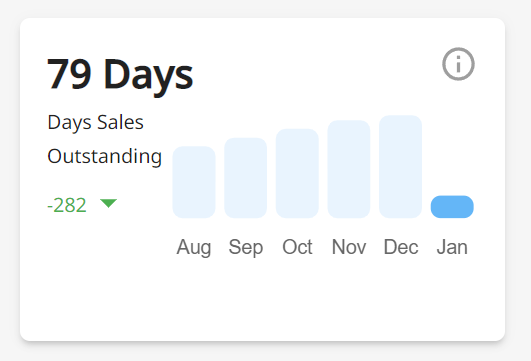Peakflo calculates your DSO using the following formula: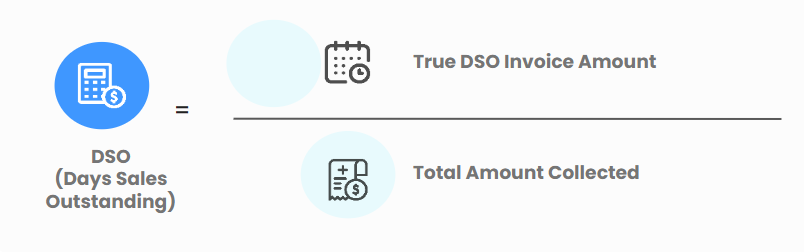First, we pull the data for all paid and unpaid invoices from today until the last 365 days. This calculation helps us better estimate the trends for DSO.

Then, we calculate the total of your invoice value. This will be the sum of:

Paid invoices: (Paid On Date - Issue Date) x Total Amount
Unpaid or overdue invoices: (Today - Issue Date) x Total Amount

The result of the total invoice value is what we call the True DSO Invoice Amount

Afterward, we divide True DSO by the total amount of revenue collected. This will then become the DSO number that you see in our dashboard!

• Doubtful debt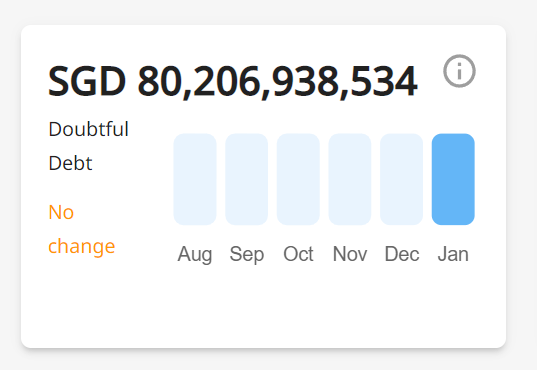This section shows the total invoice amount that is 180 days past the due date and likely to turn into bad debt.
• Outstanding amount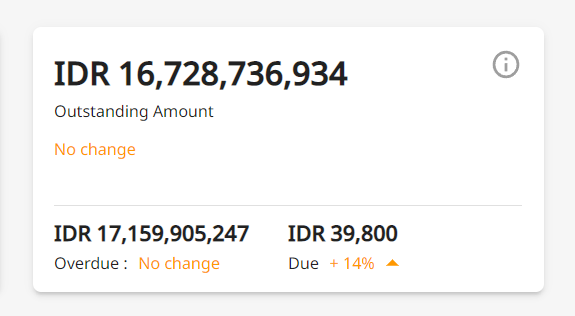This metric is calculated by the following formula: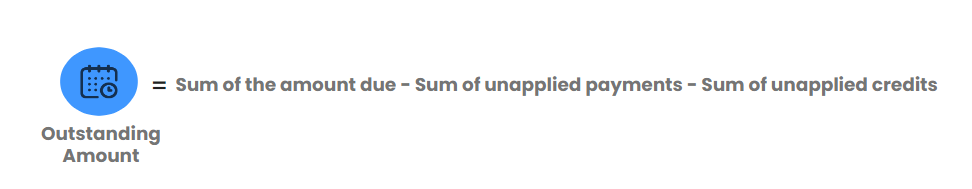In the same box, you can also find the amount overdue and the amount due.
Here's what they signify:
Due = sum of all amount due for invoices with due date in future
Overdue = sum of all amount due for invoices with due date in past
• Invoices disputed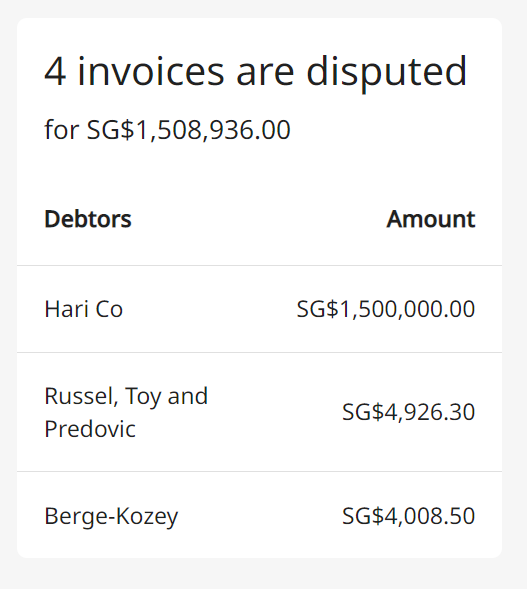A rundown of disputed invoices complete with the debtors and amount so you can resolve disputes quickly.
• Aging balanceThis chart visualizes the total outstanding amount
• 0-30 days overdue: shows the amount that is overdue less than 30 days;
• 30-60 days overdue: shows the amount that is overdue between 30-60 days;
• 60-90 days overdue: shows the amount that is overdue between 60-90 days;
• >90 days overdue: shows the amount that is overdue more than 90 days;
• Main Debtors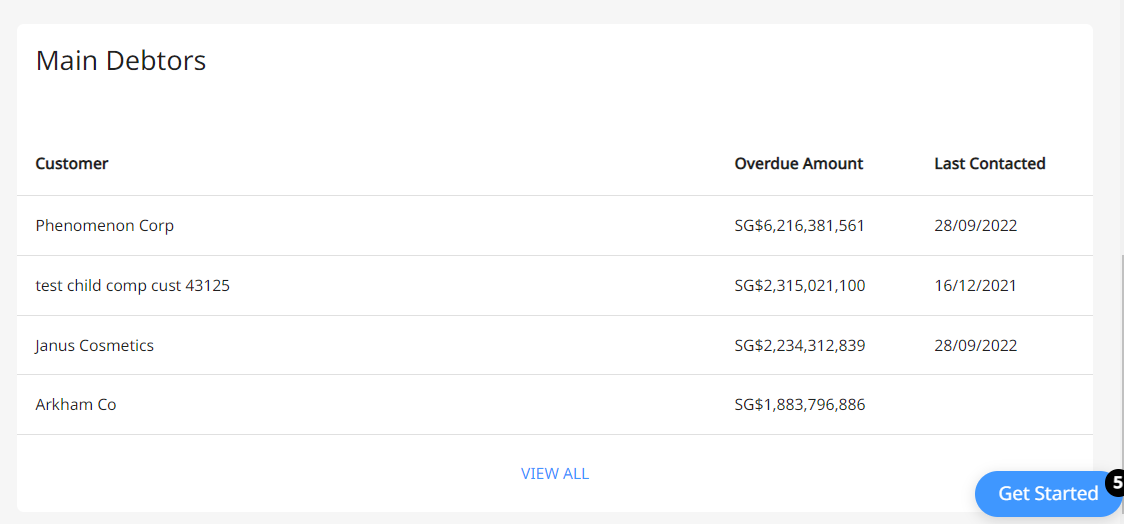These are the customers who owe you the most money.